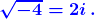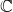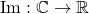# WitCH 2

Well, WitCH 1 is still not satisfactorily resolved, and Tweel’s puzzle is also still out there. But, we may as well get another ball rolling.

The second in our What is this Crap Here series comes from Cambridge’s textbook Specialist Mathematics VCE Units 3 & 4 (2018). Enjoy, and please get to pondering, and posting.## Update

Thanks to Damo for their hard work below.

The main problem with the above excerpt is that it should not exist. It is pointless to introduce complex numbers with more than a sentence on complex roots, and it is almost impossible to do so in a sensible manner. The nonsense of the text’s approach is encapsulated by the equationThis equation is best thought as false and, in the context of the excerpt above, must be thought of as meaningless. As is, thus, the discussion leading up to this equation.

How did they get there? To begin, i is introduced as a number for which i2 = -1, which is fine and good at the school level. Then, they note that the equation x2 = -1 has the two solutions i and -i, which is significantly less fine; since general complex numbers, and -i in particular, have not yet been defined, the notation -i is thus far meaningless, as is the notion of squaring this number. Still, if the sentence were more carefully worded, it would be reasonable in an introductory paragraph. The cavalier attitude to definition and meaning, however, is the sign of much worse to come.

The text continues by “declaring” that √(-1) = i, and then heads on its merry calculating way. But the calculation is complete fantasy. The declaration amounts to a (bad) definition of a specific root which cannot, in and of itself, tell us what any other root means or how it might be manipulated. So, √(-4) is as yet undefined, and the manipulation of this quantity is unjustified, as yet unjustifiable, and is best thought of as wrong.

In the real context we use √x to distinguish the positive root but it is fundamental that complex roots are multiple-valued. And, for the polynomial focus of VCE mathematics, multiple values are perfectly fine and perfectly natural. The quadratic formula remains true without change and the purportedly troublesome identityis always true (modulo the understanding that if x is a positive real then√x is now ambiguous). Moreover, with this natural interpretation, the text’s declaration that √(-1) = i is false, as is the equation √(-4) = 2i.

Admittedly, at some point it is valuable, and essential, to introduce principal values of roots, by which the text’s equation can be interpreted to be true. But principle roots are intrinsically awkward, must be introduced with great care and should only be introduced when there is a purpose. Which is not on page 1 of a school text, and arguably not ever in a school text.

Apart form the utter pointlessness and utter meaningless of the excerpt, we note:

• The text conflates the introduction of imaginary numbers in the 16th century with the introduction of the symbol i in the 18th century.
• The text implies 0 is an imaginary number, which is ok though a little peculiar.
• The real numbers and imaginary numbers are not subsets of.
• The characterisationsandare grandiose and pointless.

## 20 Replies to “WitCH 2”

1.Number 8 says:

I will admit to using this book but never having seen this glaring issue!

Defining i=sqrt(-1) is quite different to defining i such that i^2=-1.

The +,- just adds to the sense that the author is confused. I’m hesitant to blame the author completely though as this may have been something insisted upon by the publisher or possibly even a reviewer… (or, even possibly taken from a VCAA curriculum document directly)

1.marty says:

Thanks, Number 8. Yes, falsely declaring i = √(-1) is where the rot begins, though not where it ends.

2.Number 8 says:

Like sqrt(2) as a decimal in WitCH 1, it never really ends…

3.Potii says:

Don’t you refer to a and b as the real part and imaginary parts of a complex number? Not real and imaginary numbers. So bi would still be a complex number but the real part being 0 and not written.

On a side note, could you say it as no real part if a=0? I guess on the complex plane it 0 has a position and maybe you cannot say it has no real part. However, I do remember using that phrase at uni. So I’m not sure.

1.marty says:

Hi Potii. Both are acceptable. So, for the complex number 3 + 4i, 3 is the real part and 4 is the imaginary part, but it’s still ok to say 4i is an imaginary number. In fact that expression jars with me; i’m used to “imaginary” as being a synonym for complex, and I would refer to 4i as purely imaginary in that context. But the textbook’s usage appears to be common. I don’t quite understand your second point, though note that the number 0 is both real and imaginary.

1.Potii says:

You answered my second part with 4i being said to be purely imaginary. That’s just what I was wondering, that you can say there is no real part if only there is only 4i or -13i.

4.Number 8 says:

The mapping of C to R is really getting to me on this page. Like – who cares?!? Plus, since Re and Im are not functions, it smells of lacking understanding…

1.marty says:

Thanks, Number 8. Well, Re and Im *can* be thought of as functions as the text has written. (As can modulus and conjugate and argument and pretty much everything that can be interpreted in an input-output manner.) But your underlying point is absolutely correct, that it is utterly pointless and distracting to think of Re and Im in this manner in this context. It is just being pompously formal and gratuitously obfuscating, in a manner that Cambridge has turned into an art form.

5.Potii says:

Weren’t imaginary numbers introduced before the 18th century? I remember reading about roots of negative numbers coming up when solving cubics. A quick internet search says that Heron of Alexandria knew about them and Rafael Bombelli created rules for multiplication with complex numbers. Both of whom lived before the 18th century.

1.marty says:

Yes, you’re correct. The application of imaginary/complex numbers began in the 16th century, with Cardano and Bombelli. In an earlier passage, the Cambridge text briefly and badly refers to Cardano’s work.

The Cambridge passage excerpted above is not exactly wrong in the manner you suggest but it is close; it is vague, muddled and just short of meaningless. The wording confuses the introduction of the number we now denote by i with the introduction of notation for that number, and specifically the introduction of the symbol i. It is true, however, that “mathematicians” first used i in this way in the 18th century, where mathematicians = Euler and 18th century = 1777. (Other symbols were used beginning around 1760, but not to my knowledge much earlier than that.)

6.Number 8 says:

Is it wrong to “define” Re(z)=a and Im(z)=b? Is this not a bit backwards? Aren’t these labels, rather than definitions?

1.marty says:

I wouldn’t say “wrong”, but yes, it’s clumsy. Probably better to say a is defined to be the real part of z, and is denoted by Re(z).

7.Luke B says:

I noticed a small error which helped reveal a much larger mistake.
In the “Note” which discusses sqrt(a x b), I think they have neglected some values of a and b. It should hold for the real numbers a and b, where a>=0 and b>=0.

This led me to a larger error.

The author’s explain here that this is the only time that we can say sqrt(ab)=sqrt(a)*sqrt(b). However, they have disregarded this when they a moment earlier state that sqrt(4*-1)=sqrt(4)*sqrt(-1).

By defining i as i^2=-1, then we are instead able to say that sqrt(4*i^2)=sqrt(4)*sqrt(i^2) without having to contradict ourselves in the next sentence.

Am I on the right track for this Witch?

1.marty says:

Definitely the right track. I’ll reply in some detail later.

1.marty says:

Luke, you have nailed one of the major errors in the excerpt. There is no indication in the text, for any z and w, why the equation

√(zw) = √z x √w

might or might not be true.

To be fair, the text never states that this equation only holds for z and w positive [by which they mean nonnegative] real numbers. The text only states that the equation fails to hold for z and w both negative.

But, the text gives no indication why the equation should hold for z negative and w positive. Underlying this is the fundamental question: what does √z mean?

1.Damo says:

What does √z mean? A square root of z is a number w such that w^2=z. z will always have two square roots w and -w. For a positive real number z we are (somewhat) familiar with referring to the square roots of z as being √z and -√z, where √z is a positive real number and is called the principal square root, while -√z is a negative real number. With this understanding, it makes sense that √(4*9)=√4*√9=6. However, to make sense of the square roots of a negative real number z, we need to think of z as being a complex number. They appear to be assuming that √z refers to a principal square root in the same way that it does with real numbers, but they haven’t considered what the principal square root of a complex number is, which makes the whole thing kind of meaningless. One way of thinking about √(zw)=√z x √w : if a is a square root of z and b is a square root of w, then ab is a square root of zw. So, for √(-4 x -1) = √(-4) x √(-1) : if you multiply a square root of -4 by a square root of -1 you get a square root of 4. Which is true.

1.marty says:

Damo, I couldn’t have said it better myself (but I’ll try).

8.Red Five says:

I suppose you *could* get into principal roots which goes a fair bit beyond square roots, but surely there is an easier way to just avoid all this mess by using a more considered original definition for the number i?

(I’m not even pretending to be an expert, so this is a genuine question raised as an act of genuine curiosity, not making a suggestion as to how it could be done, because I’m sure my attempt would also be problematic)

1.marty says:

Thanks, RF. Hilariously, the text *did* start with a considered definition: it began with i2 = -1, which is exactly where one should begin. There was then simply no need to mention square roots at all. Not on Page 1.

As to how to define roots if one must/desires, your non-expert pondering makes a lot more sense than the text. Yes principal roots is one natural approach. The other natural approach, which Damo mentions above, is to consider √z to be double-valued. For solving polynomial equations and the like, the latter approach suffices and is a lot simpler.

1.Red Five says:

Fair point. I just noticed the other day that with cube roots of negatives, Wolfram chooses the complex number with the real imaginary part as the default answer (unless the full solution set is asked for) in a number of cases – although considering some high schools are using this program, I did wonder how many teachers would be able to trouble-shoot such a situation…

…then I opened another bottle of wine and forgot about most other things for a while.

This site uses Akismet to reduce spam. Learn how your comment data is processed.